## Engineering-Mathematics

 Question 1

If a random variable X has a Poisson distribution with mean 5, then the expectation E[(X + 2)2] equals _________.

 A 54 B 55 C 56 D 57
Engineering-Mathematics       Probability       GATE 2017 [Set-2]       Video-Explanation
Question 1 Explanation:
In Poisson distribution:
Mean = Variance
E(X) = E(X2) – (E(X))2 = 5
E(X2) = 5 + (E(X))2 = 5 + 25 = 30
So, E[(X + 2)2] = E[X2 + 4 + 4X]
= E(X2) + 4 + 4E(X)
= 30 + 4 + 4 × 5
= 54
 Question 2

If the ordinary generating function of a sequenceis, then a3 – a0 is equal to ________.

 A 15 B 16 C 17 D 18
Engineering-Mathematics       Combinatorics       GATE 2017 [Set-2]       Video-Explanation
Question 2 Explanation:Question 3A 1 B Limit does not exist C 53/12 D 108/7
Engineering-Mathematics       Calculus       GATE 2019       Video-Explanation
Question 3 Explanation:Question 4
Let G be an arbitrary group. Consider the following relations on G:
R: ∀a,b ∈ G, aRb if and only if ∃g ∈ G such that a = gbg
R: ∀a,b ∈ G, aRb if and only if a = b-1
Which of the above is/are equivalence relation/relations?
 A R2 only B R1 and R2 C Neither R1 and R2 D R1 only
Engineering-Mathematics       Set-Theory       GATE 2019       Video-Explanation
Question 4 Explanation:
A relation between the elements of a set is symmetric, reflexive and transitive then such relation is called as equivalence relation.
Consider Statement R1:
Reflexive:
aR1a
⇒ a = g-1ag
Left multiply both sides by g
⇒ ga = gg-1ag
Right multiply both sides by g-1
⇒ gag-1 = gg-1agg-1
⇒ gag-1 = a [∴ The relation is reflexive]
Symmetric:
If aR1b, then ∃g ∈ G such that gag-1 = b then a = g-1bg, which is Correct.
⇒ So, given relation is symmetric.
Transitive:
The given relation is Transitive.
So, the given relation R1 is equivalence.
R2:
The given relation is not reflexive.
So, which is not equivalence relation.
Such that a ≠ a-1.
So, only R1 is true.
 Question 5
Let X be a square matrix. Consider the following two statements on X.
I. X is invertible.
II. Determinant of X is non-zero.
Which one of the following is TRUE?
 A I implies II; II does not imply I. B II implies I; I does not imply II. C I and II are equivalent statements. D I does not imply II; II does not imply I.
Engineering-Mathematics       Linear-Algebra       GATE 2019       Video-Explanation
Question 5 Explanation:
X is invertible means, that X is non-singular matrix.
That means we can also say that determinant of X is non-zero.
 Question 6

Let G be an undirected complete graph on n vertices, where n > 2. Then, the number of different Hamiltonian cycles in G is equal to

 A n! B 1 C (n-1)! D
Engineering-Mathematics       Graph-Theory       GATE 2019       Video-Explanation
Question 6 Explanation:
A Hamiltonian cycle is a closed loop on a graph where every node (vertex) is visited exactly once.
The total number of hamiltonian cycles in a complete graph are
(n-1)!/2, where n is number of vertices.
 Question 7
Let U = {1,2,…,n}. Let A = {(x,X)|x ∈ X, X ⊆ U}. Consider the following two statements on |A|.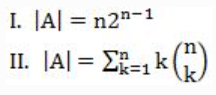Which of the above statements is/are TRUE?
 A Only II B Only I C Neither I nor II D Both I and II
Engineering-Mathematics       Set-Theory       GATE 2019       Video-Explanation
Question 7 Explanation:
Let us consider U = {1, 2}
and given A = {(x, X), x∈X and X⊆U}
Possible sets for U = {Φ, {1}, {2}, {1, 2}}
if x=1 then no. of possible sets = 2
x=2 then no. of possible sets = 2
⇒ No. of possible sets for A = (no. of sets at x=1) + (no. of sets at x=2) = 2 + 2 = 4
Consider statement (i) & (ii) and put n=2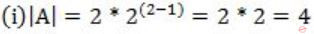Statement (i) is true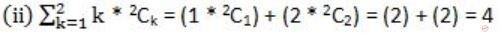Statement (i) and (ii) both are true.
Video Explanation
 Question 8

Suppose Y is distributed uniformly in the open interval (1,6). The probability that the polynomial 3x2 + 6xY + 3Y + 6 has only real roots is (rounded off to 1 decimal place) _____.

 A 0.3 B 0.9 C 0.1 D 0.8
Engineering-Mathematics       Probability       GATE 2019       Video-Explanation
Question 8 Explanation:
Given polynomial equation is
3x2 + 6xY + 3Y + 6
= 3x2 + (6Y)x + (3Y + 6)
which is in the form: ax2 + bx + c
For real roots: b2 – 4ac ≥ 0
⇒ (6Y)2 – 4(3)(3Y + 6) ≥ 0
⇒ 36Y2 – 36Y – 72 ≥ 0
⇒ Y2 – Y – 2 ≥ 0
⇒ (Y+1)(Y-2) ≥ 0
Y = -1 (or) 2
The given interval is (1,6).
So, we need to consider the range (2,6).
The probability = (1/(6-1)) * (6-2) = 1/5 * 4 = 0.8
 Question 9

Consider the first order predicate formula φ:

∀x[(∀z z|x ⇒ ((z = x) ∨ (z = 1))) ⇒ ∃w (w > x) ∧ (∀z z|w ⇒ ((w = z) ∨ (z = 1)))]

Here ‘a|b’ denotes that ‘a divides b’, where a and b are integers. Consider the following sets:

S1.  {1, 2, 3, …, 100}
S2.  Set of all positive integers
S3. Set of all integers

Which of the above sets satisfy φ?

 A S1 and S3 B S1, S2 and S3 C S2 and S3 D S1 and S2
Engineering-Mathematics       Propositional-Logic       GATE 2019       Video-Explanation
Question 9 Explanation:
The first order logic gives the meaning that if z is a prime number then there exists another prime number in the set which is larger than it.
One of the case:
If -7 is a number which is prime (either divided by -7 or 1 only). then there exists some number like -3 which is larger than -7 also satisfy the property (either divided by -3 or 1 only).
So, S3 is correct
It’s true for all integers too.
 Question 10

Consider the following matrix: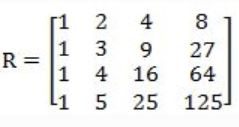The absolute value of the product of Eigen values of R is ______.

 A 12 B 17 C 10 D 8
Engineering-Mathematics       Linear-Algebra       GATE 2019       Video-Explanation
Question 10 Explanation:Question 11The largest eigenvalue of A is ________

 A 3 B 4 C 5 D 6
Engineering-Mathematics       Linear-Algebra       GATE 2018       Video-Explanation
Question 11 Explanation:Correction in Explanation: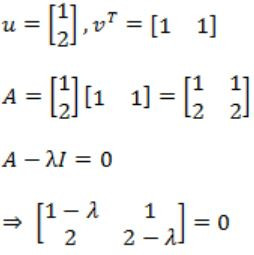⇒ (1 – λ)(2 – λ) – 2 = 0
⇒ λ2 – 3λ=0
λ = 0, 3
So maximum is 3.
 Question 12

Two people, P and Q, decide to independently roll two identical dice, each with 6 faces, numbered 1 to 6. The person with the lower number wins. In case of a tie, they roll the dice repeatedly until there is no tie. Define a trial as a throw of the dice by P and Q. Assume that all 6 numbers on each dice are equi-probable and that all trials are independent. The probability (rounded to 3 decimal places) that one of them wins on the third trial is __________.

 A 0.021 B 0.022 C 0.023 D 0.024
Engineering-Mathematics       Probability       GATE 2018       Video-Explanation
Question 12 Explanation:
When two identical dice are rolled
⇾ A person wins who gets lower number compared to other person.
⇾ There could be “tie”, if they get same number.
Favorable cases = {(1, 1), (2, 2), (3, 3), (4, 4), (5, 5), (6, 6)}
Probability (tie) = 6/36 (when two dice are thrown, sample space = 6 × 6 = 36)
= 1/6
“Find the probability that one of them wins in the third attempt”
⇾ Which means, first & second time it should be tie and third time it should not be tie
⇾ P (tie) * P (tie) * P (not tie)
⇒ 1/6* 1/6 * (1 – 1/6)
⇒ (5/36×6)
= 0.138/6
= 0.023
 Question 13

The chromatic number of the following graph is _______.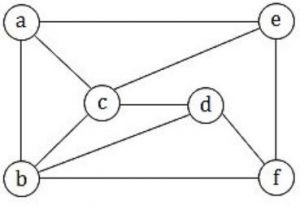A 1 B 2 C 3 D 4
Engineering-Mathematics       Graph-Theory       GATE 2018       Video-Explanation
Question 13 Explanation:
Chromatic number of the following graph is “3”Question 14

Let G be a finite group on 84 elements. The size of a largest possible proper subgroup of G is _________.

 A 41 B 42 C 43 D 44
Engineering-Mathematics       Set-Theory       GATE 2018       Video-Explanation
Question 14 Explanation:
Lagranges Theorem:
For any group ‘G’ with order ‘n’, every subgroup ‘H’ has order ‘k’ such that ‘n’ is divisible by ‘k’.
Solution:
Given order n = 84
Then the order of subgroups = {1, 2, 3, 4, 6, 7, 12, 14, 21, 28, 42, 84}
As per the proper subgroup definition, it should be “42”.
 Question 15

Which one of the following is a closed form expression for the generating function of the sequence {an}, where an = 2n+3 for all n = 0, 1, 2, …?

 A 3/(1-x)2 B 3x/(1-x)2 C 2-x/(1-x)2 D 3-x/(1-x)2
Engineering-Mathematics       Combinatorics       GATE 2018       Video-Explanation
Question 15 Explanation:Question 16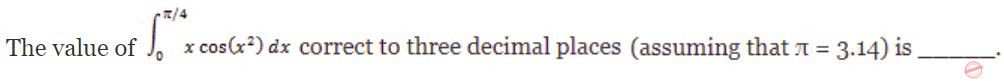A 0.289 B 0.298 C 0.28 D 0.29
Engineering-Mathematics       Calculus       GATE 2018       Video-Explanation
Question 16 Explanation: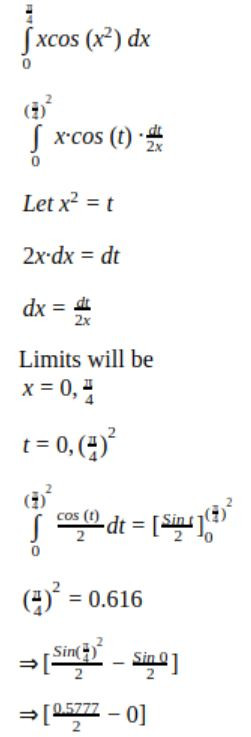Question 17

Assume that multiplying a matrix G1 of dimension p×q with another matrix G2 of dimension q×r requires pqr scalar multiplications. Computing the product of n matrices G1G2G3…Gn can be done by parenthesizing in different ways. Define GiGi+1 as an explicitly computed pair for a given parenthesization if they are directly multiplied. For example, in the matrix multiplication chain G1G2G3G4G5G6 using parenthesization(G1(G2G3))(G4(G5G6)), G2G3 and G5G6 are the only explicitly computed pairs.

Consider a matrix multiplication chain F1F2F3F4F5, where matrices F1, F2, F3, F4 and F5 are of dimensions 2×25, 25×3, 3×16, 16×1 and 1×1000, respectively. In the parenthesization of F1F2F3F4F5 that minimizes the total number of scalar multiplications, the explicitly computed pairs is/ are

 A F1F2 and F3F4 only B F2F3 only C F3F4 only D F1F2 and F4F5 only
Engineering-Mathematics       Linear-Algebra       GATE 2018       Video-Explanation
Question 17 Explanation:
→ As per above information, the total number of scalar multiplications are 2173.
→ Optimal Parenthesization is:
((F1(F2(F3 F4)))F5)
→ But according to the problem statement we are only considering F3, F4 explicitly computed pairs.
 Question 18
Consider the first-order logic sentence
φ ≡ ∃s∃t∃u∀v∀w∀x∀y ψ(s,t,u,v,w,x,y)
where ψ(s,t,u,v,w,x,y) is a quantifier-free first-order logic formula using only predicate symbols, and possibly equality, but no function symbols. Suppose φ has a model with a universe containing 7 elements.
Which one of the following statements is necessarily true?
 A There exists at least one model of φ with universe of size less than or equal to 3. B There exists no model of φ with universe of size less than or equal to 3. C There exists no model of φ with universe of size greater than 7. D Every model of φ has a universe of size equal to 7.
Engineering-Mathematics       Propositional-Logic       GATE 2018       Video-Explanation
Question 18 Explanation:
φ = ∃s∃t∃u∀v∀w∀x∀y ψ (s,t,u,v,w,x,y)
“∃” there exists quantifier decides whether a sentence belong to the model or not.
i.e., ~∃ will make it not belong to the model. (1) We have ‘7’ elements in the universe, So max. size of universe in a model = ‘7’
(2) There are three ‘∃’ quantifiers, which makes that a model have atleast “3” elements. So, min. size of universe in model = ‘7’.
(A) is False because: (2)
(B) is true
(C) is false because of (1)
(D) is false, because these all models with size {3 to 7} not only ‘7’.
 Question 19

Consider Guwahati (G) and Delhi (D) whose temperatures can be classified as high (H), medium (M) and low (L). Let P(HG) denote the probability that Guwahati has high temperature. Similarly, P(MG) and P(LG) denotes the probability of Guwahati having medium and low temperatures respectively. Similarly, we use P(HD), P(MD) and P(LD) for Delhi.

The following table gives the conditional probabilities for Delhi’s temperature given Guwahati’s temperature.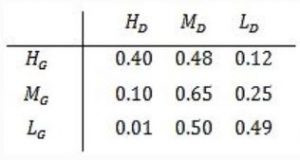Consider the first row in the table above. The first entry denotes that if Guwahati has high temperature (HG) then the probability of Delhi also having a high temperature (HD) is 0.40; i.e., P(HD ∣ HG) = 0.40. Similarly, the next two entries are P(MD ∣ HG) = 0.48 and P(LD ∣ HG) = 0.12. Similarly for the other rows.

If it is known that P(HG) = 0.2, P(MG) = 0.5, and P(LG) = 0.3, then the probability (correct to two decimal places) that Guwahati has high temperature given that Delhi has high temperature is _______ .

 A 0.6 B 0.61 C 0.62 D 0.63
Engineering-Mathematics       Linear-Algebra       GATE 2018       Video-Explanation
Question 19 Explanation: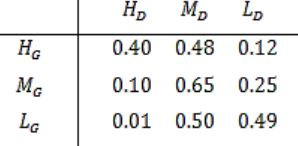The first entry denotes that if Guwahati has high temperature (HG ) then the probability that Delhi also having a high temperature (HD ) is 0.40.
P (HD / HG ) = 0.40
We need to find out the probability that Guwahati has high temperature.
Given that Delhi has high temperature (P(HG / HD )).P (HD / HG ) = P(HG ∩ HD ) / P(HD )
= 0.2×0.4 / 0.2×0.4+0.5×0.1+0.3×0.01
= 0.60
 Question 20
Let N be the set of natural numbers. Consider the following sets,
P: Set of Rational numbers (positive and negative)
Q: Set of functions from {0, 1} to N
R: Set of functions from N to {0, 1}
S: Set of finite subsets of N
Which of the above sets are countable?
 A Q and S only B P and S only C P and R only D P, Q and S only
Engineering-Mathematics       Linear-Algebra       GATE 2018       Video-Explanation
Question 20 Explanation:
Set of rational numbers are countable. It is proved by various methods in literature.
Set of functions from {0,1} to N is countable as it has one to one correspondence to N.
Set of functions from N to {0,1} is uncountable, as it has one to one correspondence to set of real numbers between (0 and 1).
Set of finite subsets of N is countable.
 Question 21
Consider a matrix P whose only eigenvectors are the multiples of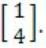.
Consider the following statements.
(I)P does not have an inverse
(II)P has a repeated eigenvalue
(III)P cannot be diagonalized
Which one of the following options is correct?
 A Only I and III are necessarily true B Only II is necessarily true C Only I and II are necessarily true D Only II and III are necessarily true
Engineering-Mathematics       Linear-Algebra       GATE 2018       Video-Explanation
Question 21 Explanation:Though the multiple of a vector represents same vector, and each eigen vector has distinct eigen value, we can conclude that ‘p’ has repeated eigen value.
If the unique eigen value corresponds to an eigen vector e, but the repeated eigen value corresponds to an entire plane, then the matrix can be diagonalized, using ‘e’ together with any two vectors that lie in plane.
But, if all eigen values are repeated, then the matrix cannot be diagonalized unless it is already diagonal.
So (III) holds correct.
A diagonal matrix can have inverse, So (I) is false.
Then (II) and (III) are necessarily True.
 Question 22

Let G be a graph with 100! vertices, with each vertex labeled by a distinct permutation of the numbers 1, 2, …, 100. There is an edge between vertices u and v if and only if the label of u can be obtained by swapping two adjacent numbers in the label of v. Let y denote the degree of a vertex in G, and z denote the number of connected components in G.

Then, y + 10z = ___________.

 A 109 B 110 C 111 D 112
Engineering-Mathematics       Graph-Theory       GATE 2018       Video-Explanation
Question 22 Explanation:
G is a graph with 100! vertices. Label of each vertex obtains from distinct permutation of numbers “1, 2, … 100”.
There exists edge between two vertices iff label of ‘u’ is obtained by swapping two adjacent numbers in label of ‘v’.
Example:
12 & 21, 23 & 34
The sets of the swapping numbers be (1, 2) (2, 3) (3, 4) … (99).
The no. of such sets are 99 i.e., no. of edges = 99.
As this is regular, each vertex has ‘99’ edges correspond to it.
So degree of each vertex = 99 = y.
As the vertices are connected together, the number of components formed = 1 = z
y + 102 = 99 + 10(1) = 109
 Question 23
The statement (¬p) ⇒ (¬q) is logically equivalent to which of the statements below?
I. p ⇒ q
II. q ⇒ p
III. (¬q) ∨ p
IV. (¬p) ∨ q
 A I only B I and IV only C II only D II and III only
Engineering-Mathematics       Propositional-Logic       GATE 2017 [Set-1]       Video-Explanation
Question 23 Explanation:
Method 1:
Construct Truth tables:
~p ⇒ ~q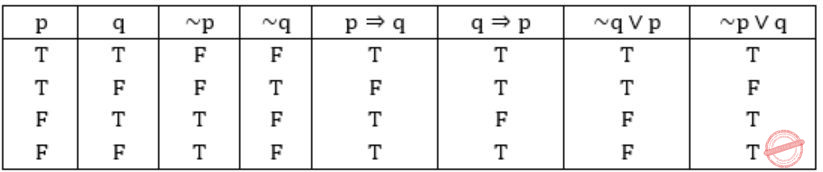II, III are equivalent to (~p) ⇒ (~q)
Method 2:
(I) p⇒q ≡ ~p∨q
(II) q⇒p ≡ ~q∨p
(III) (~q) ∨ p ≡ ~q∨p
(IV) (~p) ∨ p ≡ ~p∨q
Also, from question:
(~p) ⇒ (~q)
≡ p∨~q
So, (II) & (III) are equivalent to the statement given in question.
 Question 24
Consider the first-order logic sentence F: ∀x(∃yR(x,y)). Assuming non-empty logical domains, which of the sentences below are implied by F?
I. ∃y(∃xR(x,y))
II. ∃y(∀xR(x,y))
III. ∀y(∃xR(x,y))
IV. ¬∃x(∀y¬R(x,y))
 A IV only B I and IV only C II only D II and III only
Engineering-Mathematics       Prepositional-Logic       GATE 2017 [Set-1]       Video-Explanation
Question 24 Explanation:
Lets convert the given first order logic sentence into some english sentence.
F: ∀x(∃yR(x,y)) (given)
: For all girls there exist a boyfriend
(x for girls and y for boys)
I: ∃y(∃xR(x,y))
: There exist some boys who have girlfriends.
(Subset of statement F, so True)
II: ∃y(∀xR(x,y))
: There exists some boys for which all the girls are girlfriend. (False)
III: ∀y(∃xR(x,y))
: For all boys exists a girlfriend. (False)
IV: ~∃x(∀y~R(x,y))
= ∀x(~∀y~R(x,y))
= ∀x(∃yR(x,y)) (∵ ~∀y=∃y, ~∃x=∀x)
(True)
 Question 25

Let c1, cn be scalars not all zero. Such that the following expression holds:where ai is column vectors in Rn. Consider the set of linear equations.

Ax = B.

where A = [a1…….an] andThen, Set of equations has

 A a unique solution at x = Jn where Jn denotes a n-dimensional vector of all 1 B no solution C infinitely many solutions D finitely many solutions
Engineering-Mathematics       Linear-Algebra       GATE 2017 [Set-1]       Video-Explanation
Question 25 Explanation:
ai is a column vector
AX = BAs given thatand c1&cn ≠ 0
means c0a0 + c1a1 + …cnan = 0, represents that a0, a1… an are linearly dependent.
So rank of ‘A’ = 0, (so if ‘B’ rank is = 0 infinite solution, ‘B’ rank>0 no solution) ⇾(1)
Another condition given here is, ‘Σai = b’,
so for c1c2…cn = {1,1,…1} set, it is having value ‘b’,
so there exists a solution if AX = b →(2)
From (1)&(2), we can conclude that AX = B has infinitely many solutions.
 Question 26

Let T be a binary search tree with 15 nodes. The minimum and maximum possible heights of T are:

Note: The height of a tree with a single node is 0.
 A 4 and 15 respectively B 3 and 14 respectively C 4 and 14 respectively D 3 and 15 respectively
Engineering-Mathematics       Binary-Trees       GATE 2017 [Set-1]       Video-Explanation
Question 26 Explanation:
T be a Binary Search Tree with 15 nodes.
The height of a tree with single node is 0.
Minimum possible height is when it is a complete binary tree.Maximum possible height is when it is a skewed tree left/right.So the minimum and maximum possible heights of T are: 3 and 14 respectively.
 Question 27

Let X be a Gaussian random variable with mean 0 and variance σ2. Let Y = max(X, 0) where max(a,b) is the maximum of a and b. The median of Y is __________.

 A 0 B 1 C 2 D 3
Engineering-Mathematics       Probability       GATE 2017 [Set-1]       Video-Explanation
Question 27 Explanation:
Given that, Y = max(X,0),which means, Y is 0 for negative values of X and Y is X for positive values of X.
Median is a point, where the probability of getting less than median is 1/2 and probability of getting greater than median is 1/2.
From the given details, we can simply conclude that, median is 0. (0 lies exactly between positive and negative values)Question 28
The value ofA is 0 B is -1 C is 1 D does not exist
Engineering-Mathematics       Calculus       GATE 2017 [Set-1]       Video-Explanation
Question 28 Explanation:If “x=1” is substituted we get 0/0 form, so apply L-Hospital ruleSubstitute x=1
⇒ (7(1)6-10(1)4)/(3(1)2-6(1)) = (7-10)/(3-6) = (-3)/(-3) = 1Question 29

Let p, q and r be prepositions and the expression (p → q) → r be a contradiction. Then, the expression (r → p) → q is

 A a tautology B a contradiction C always TRUE when p is FALSE D always TRUE when q is TRUE
Engineering-Mathematics       Prepositional-Logic       GATE 2017 [Set-1]       Video-Explanation
Question 29 Explanation:
Given that (p→q)→r is a contradiction.
So r = F and (p→q) = T.
We have to evaluate the expression
(r→p)→q
Since r = F, (r→p) = T (As F→p, is always true)
The final expression is T→q and this is true when q is true, hence option D.
 Question 30

Let u and v be two vectors in R2 whose Euclidean norms satisfy ||u||=2||v||. What is the value of α such that w = u + αv bisects the angle between u and v?

 A 2 B 1/2 C 1 D -1/2
Engineering-Mathematics       Linear-Algebra       GATE 2017 [Set-1]       Video-Explanation
Question 30 Explanation:Let u, v be vectors in R2, increasing at a point, with an angle θ.
A vector bisecting the angle should split θ into θ/2, θ/2
Means ‘w’ should have the same angle with u, v and it should be half of the angle between u and v.
Assume that the angle between u, v be 2θ (thus angle between u,w = θ and v,w = θ)
Cosθ = (u∙w)/(∥u∥ ∥w∥) ⇾(1)
Cosθ = (v∙w)/(∥v∥ ∥w∥) ⇾(2)
(1)/(2) ⇒ 1/1 = ((u∙w)/(∥u∥ ∥w∥))/((v∙w)/(∥v∥ ∥w∥)) ⇒ 1 = ((u∙w)/(∥u∥))/((v∙w)/(∥v∥))
⇒ (u∙w)/(v∙w) = (∥u∥)/(∥v∥) which is given that ∥u∥ = 2 ∥v∥
⇒ (u∙w)/(v∙w) = (2∥v∥)/(∥v∥) = 2 ⇾(3)
Given ∥u∥ = 2∥v∥
u∙v = ∥u∥ ∥v∥Cosθ
=2∙∥v∥2 Cosθ
w = u+αv
(u∙w)/(v∙w) = 2
(u∙(u+αv))/(v∙(u+αv)) = 2
(u∙u+α∙u∙v)/(u∙v+α∙v∙v) = 2a∙a = ∥a∥2
4∥v∥2+α∙2∙∥v∥2 Cosθ = 2(2∥v∥2 Cosθ+α∙∥v∥2)
4+2αCosθ = 2(2Cosθ+α)
4+2αCosθ = 4Cosθ+2α ⇒ Cosθ(u-v)+2α-4 = 0
4-2α = Cosθ(4-2α)
(4-2α)(Cosθ-1) = 0
4-2α = 0Question 31
Let A be m×n real valued square symmetric matrix of rank 2 with expression given below.Consider the following statements
(i) One eigenvalue must be in [-5, 5].
(ii) The eigenvalue with the largest magnitude must be strictly greater than 5.
Which of the above statements about eigenvalues of A is/are necessarily CORRECT?
 A Both (I) and (II) B (I) only C (II) only D Neither (I) nor (II)
Engineering-Mathematics       Linear-Algebra       GATE 2017 [Set-1]       Video-Explanation
Question 31 Explanation:
Letbe a real valued, rank = 2 matrix.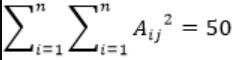a2+b2+c2+d2 = 50
Square values are of order 0, 1, 4, 9, 16, 25, 36, …
So consider (0, 0, 5, 5) then Sum of this square = 0+0+25+25=50
To get rank 2, the 2×2 matrix can beThe eigen values are,
|A-λI| = 0 (The characteristic equation)-λ(-λ)-25 = 0
λ2-25 = 0So, the eigen values are within [-5, 5], Statement I is correct.
The eigen values with largest magnitude must be strictly greater than 5: False.
So, only Statement I is correct.
 Question 32

The number of integers between 1 and 500 (both inclusive) that are divisible by 3 or 5 or 7 is _________.

 A 271 B 272 C 273 D 274
Engineering-Mathematics       Combinatorics       GATE 2017 [Set-1]       Video-Explanation
Question 32 Explanation: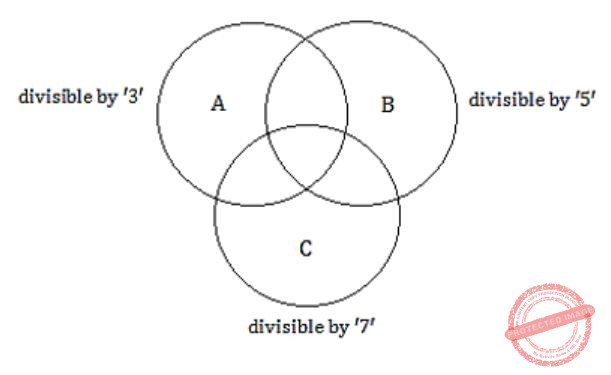Let A = number divisible by 3
B = numbers divisible by 5
C = number divisible by 7
We need to find “The number of integers between 1 and 500 that are divisible by 3 or 5 or 7″ i.e., |A∪B∪C|
We know,
|A∪B∪C| = |A|+|B|+C-|A∩B|-|A∩C|-|B∩C|+|A∩B|
|A| = number of integers divisible by 3
[500/3 = 166.6 ≈ 166 = 166]
|B| = 100
[500/5 = 100]
|C| = 71
[500/7 = 71.42]
|A∩B| = number of integers divisible by both 3 and 5 we need to compute with LCM (15)
i.e.,⌊500/15⌋ ≈ 33
|A∩B| = 33
|A∩C| = 500/LCM(3,7) 500/21 = 23.8 ≈ 28
|B∩C| = 500/LCM(5,3) = 500/35 = 14.48 ≈ 14
|A∩B∩C| = 500/LCM(3,5,7) = 500/163 = 4.76 ≈ 4
|A∪B∪C| = |A|+|B|+|C|-|A∩B|-|A∩C|-|B∩C|+|A∩B∩C|
= 166+100+71-33-28-14+4
= 271
 Question 33

If f(x) = Rsin(πx/2) + S, f'(1/2) = √2 and, then the constants R and S are, respectively.

 A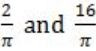B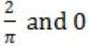C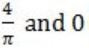DEngineering-Mathematics       Calculus       GATE 2017 [Set-2]       Video-Explanation
Question 33 Explanation: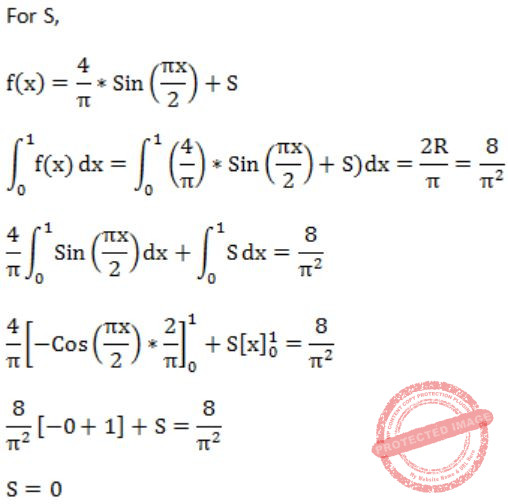Question 34

Let p, q, r denote the statements “It is raining”, “It is cold”, and “It is pleasant”, respectively. Then the statement “It is not raining and it is pleasant, and it is not pleasant only if it is raining and it is cold” is represented by

 A (¬p ∧ r) ∧ (¬r → (p ∧ q)) B (¬p ∧ r) ∧ ((p ∧ q) → ¬r) C (¬p ∧ r) ∨ ((p ∧ q) → ¬r) D (¬p ∧ r) ∨ (r → (p ∧ q))
Engineering-Mathematics       Prepositional-Logic       GATE 2017 [Set-2]       Video-Explanation
Question 34 Explanation:
p: It is raining
q: It is cold
r: It is pleasant
“If it is not raining and it is pleasant, and it is not pleasant only if it is raining and it is cold.”
We can divide the statement into two parts with “Conjunction”.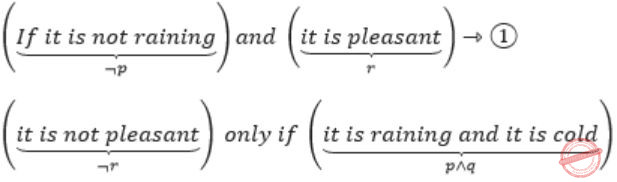i.e., ¬r→(p∧q) ⇾(2)
From (1) & (2), the given statement can be represented asQuestion 35

Consider the set X = {a,b,c,d e} under the partial ordering

R = {(a,a),(a,b),(a,c),(a,d),(a,e),(b,b),(b,c),(b,e),(c,c),(c,e),(d,d),(d,e),(e,e)}.

The Hasse diagram of the partial order (X,R) is shown below.The minimum number of ordered pairs that need to be added to R to make (X,R) a lattice is _________.

 A 0 B 1 C 2 D 3
Engineering-Mathematics       Set-Theory       GATE 2017 [Set-2]       Video-Explanation
Question 35 Explanation:
R = {(a,a), (a,b), (a,c), (a,d), (a,e), (b,b), (b,c), (b,e), (c,c), (c,e), (d,d), (d,e), (e,e)}
As per the definition of lattice, each pair should have GLB, LUB.
The given ‘R’ has GLB, LUB for each and every pair.
So, no need to add extra pair.
Thus no. of required pair such that Hasse diagram to become lattice is “0”.
 Question 36Then the rank of P+Q is _________.

 A 2 B 3 C 4 D 5
Engineering-Mathematics       Matrices       GATE 2017 [Set-2]       Video-Explanation
Question 36 Explanation:R2→R2+R1The number of non-zero rows of P + Q = 2,
So Rank = 2
Note: “Rank” is the number of independent vectors.
Method-1:
Each vector is a row in matrix.
Echelon form of a matrix has no. of zeroes increasing each rows.
The total non-zero rows left give the rank.
Method-2:
Find determinant of matrix, for 3×5, if determinant is ‘0’, the max rank can be 2.
If determinant of any n×n is non-zero, then rows proceed with (n-1)×(n-1).
 Question 37

G is an undirected graph with n vertices and 25 edges such that each vertex of G has degree at least 3. Then the maximum possible value of n is ___________.

 A 16 B 17 C 18 D 19
Engineering-Mathematics       Graph-Theory       GATE 2017 [Set-2]       Video-Explanation
Question 37 Explanation:
An undirected graph ‘G’ has ‘n’ vertices & 25 edges.
Degree of each vertex ≥ 3|v| = 2|E|
The relation between max and min degree of graph are
m ≤ 2|E| / |v| ≤ M
Given minimum degree = 3
So, 3 ≤2 |E| / |v|
3|v| ≤ 2|E|
3(n) ≤ 2(25)
n ≤ 50/3
n ≤ 16.6
(n = 16)
 Question 38

P and Q are considering to apply for job. The probability that p applies for job is 1/4. The probability that P applies for job given that Q applies for the job 1/2 and The probability that Q applies for job given that P applies for the job 1/3.The probability that P does not apply for job given that Q does not apply for the job

 A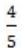BC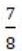DEngineering-Mathematics       Probability       GATE 2017 [Set-2]       Video-Explanation
Question 38 Explanation:
Probability that ‘P’ applies for a job = 1/4 = P(p) ⇾ (1)
Probability that ‘P’ applies for the job given that Q applies for the job = P(p/q) = 1/2 ⇾ (2)
Probability that ‘Q’ applies for the job, given that ‘P’ applies for the job = P(p/q) = 1/3 ⇾ (3)
Bayes Theorem:
(P(A/B) = (P(B/A)∙P(A))/P(B) ; P(A/B) = P(A∩B)/P(B))
⇒ P(p/q) = (P(q/p)∙P(p))/p(q)
⇒ 1/2 = (1/3×1/4)/p(q)
p(q) = 1/12×2 = 1/(6) (P(q) = 1/6) ⇾ (4)
From Bayes,
P(p/q) = (P(p∩q))/(P(q))
1/2 = P(p∩q)/(1⁄6)
(p(p∩q) = 1/12)
We need to find out the “probability that ‘P’ does not apply for the job given that q does not apply for the job = P(p’/q’)
From Bayes theorem,
P(p’/q’) = (P(p’∩q’))/P(q’) ⇾ (5)
We know,
p(A∩B) = P(A) + P(B) – P(A∪B)
also (P(A’∩B’) = 1 – P(A∪B))
P(p’∩q’) = 1 – P(p∪q)
= 1 – (P(p) + P(q) – P(p∩q))
= 1 – (P(p) + P(q) – P(p) ∙ P(q))
= 1 – (1/4 + 1/6 – 1/12)
= 1 – (10/24 – 2/24)
= 1 – (8/24)
= 2/3
(P(p’∩q’) = 2/3) ⇾ (6)
Substitute in (5),
P(p’⁄q’) = (2⁄3)/(1-P(q)) = (2⁄3)/(1-1/6) = (2⁄3)/(5⁄6) = 4/5
(P(p’/q’) = 4/5)
 Question 39

For any discrete random variable X, with probability mass function P(X=j)=pj, pj≥0, j∈{0, …, N} and, define the polynomial function. For a certain discrete random variable Y, there exists a scalar β∈[0,1] such that gy(Z)=(1-β+βz)N. The expectation of Y is

 A Nβ(1 – β) B Nβ C N(1 – β) D Not expressible in terms of N and β alone
Engineering-Mathematics       Probability       GATE 2017 [Set-2]       Video-Explanation
Question 39 Explanation:
For a discrete random variable X,
Given gy (z) = (1 – β + βz)N ⇾ it is a binomial distribution like (x+y)n
Expectation (i.e., mean) of a binomial distribution will be np.
The polynomial function,
givenMean of Binomial distribution of b(xj,n,p)=The probability Mass function,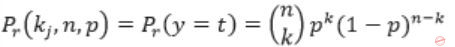Given:Question 40

If the characteristic polynomial of a 3 × 3 matrix M over R (the set of real numbers) is λ3 – 4λ2 + aλ + 30, a ∈ ℝ, and one eigenvalue of M is 2, then the largest among the absolute values of the eigenvalues of M is ________.

 A 5 B 6 C 7 D 8
Engineering-Mathematics       Linear-Algebra       GATE 2017 [Set-2]       Video-Explanation
Question 40 Explanation:
For a 3 × 3 matrix ‘M’, the characteristic equation |A – λI| is
λ3 – 4λ2 + aλ + 30 = 0 ⇾ (1)
One eigen value is ‘2’, so substitute it
23 – 4(2)2 + a(2) + 30 = 0
8 – 16 + 2a + 30 = 0
2a = -22
a = -11
Substitute in (1),
λ3 – 4λ2 – 11 + 30 = 0So, (1) can be written as
(λ – 2)(λ2 – 2λ – 15) = 0
(λ – 2)(λ2 – 5λ + 3λ – 15) = 0
(λ – 2)(λ – 3)(λ – 5) = 0
λ = 2, 3, 5
Max λ=5
 Question 41
Let p,q,r,s represent the following propositions.
p: x ∈ {8,9,10,11,12}
q: x is a composite number
r: x is a perfect square<
s: x is a prime number
The integer x≥2 which satisﬁes ¬((p ⇒ q) ∧ (¬r ∨ ¬s))  is _________.
 A 11 B 12 C 13 D 14
Engineering-Mathematics       Prepositional-Logic       GATE 2016 [Set-1]       Video-Explanation
Question 41 Explanation:
Given,
~((p→q) ∧ (~r ∨ ~S))
⇒ first simplify the given statement by converging them to ∧, ∨
⇒ [~(p→q) ∨ (~(~r ∨ ~s)]
Demorgan’s law:
⇒ [~(~p ∨ q) ∨ (r ∧ s)]
∵ p→q ≡ ~p ∨ q
⇒ [(p ∧ ~q) ∨ (r ∧ s)]
p ∧ ~q is {8,9,10,11,12} ∧ {not a composite number} i.e. {11}
r ∧ s is {perfect square} ∧ {prime} i.e. no answer
So, the one and only answer is 11.
 Question 42

Let an be the number of n-bit strings that do NOT contain two consecutive 1s. Which one of the following is the recurrence relation for an?

 A an = a(n-1) + 2a(n-2) B an = a(n-1) + a(n-2) C an = 2a(n-1) + a(n-2) D an = 2a(n-1) + 2a(n-2)
Engineering-Mathematics       Combinatorics       GATE 2016 [Set-1]       Video-Explanation
Question 42 Explanation:
an = number of n-bit strings, that do not have two consecutive 1’s.
If n=1, we have {0,1}
# Occurrences = 2
If n=2, we have {00,01,10}
# Occurrences = 3
If n=3, we have {000,001,010,100,101}
# Occurrences = 5
It is evident that a3 = a1 + a2
Similarly, an = an-1 + an-2
 Question 43A 4 B 3 C 2 D 1
Engineering-Mathematics       Calculus       GATE 2016 [Set-1]       Video-Explanation
Question 43 Explanation:Question 44

A probability density function on the interval [a,1] is given by 1/x2 and outside this interval the value of the function is zero. The value of a is _________.

 A 0.7 B 0.6 C 0.5 D 0.8
Engineering-Mathematics       Probability       GATE 2016 [Set-1]       Video-Explanation
Question 44 Explanation:
The property of probability density function is area under curve = 1
or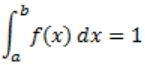where (a, b) is internal and f(x) is probability density function.
Given,
f(x) = 1/x2 , a≤x≤1
The area under curve,– 1 + 1/a = 1
1/a = 2
a = 0.5
 Question 45

Two eigenvalues of a 3 × 3 real matrix P are (2 + √-1) and 3. The determinant of P is __________.

 A 18 B 15 C 17 D 16
Engineering-Mathematics       Linear-Algebra       GATE 2016 [Set-1]       Video-Explanation
Question 45 Explanation:
If an eigen value of a matrix is a complex number, then there will be other eigen value, which is conjugate of the complex eigen value.
So, For the given 3×3 matrix there would be 3 eigen values.
Given eigen values are : 2+i and 3.
So the third eigen value should be 2-i.
As per the theorems, the determinant of the matrix is the product of the eigen values.
So the determinant is (2+i)*(2-i)*3 = 15.
 Question 46

The coefﬁcient of x12 in (x3 + x4 + x5 + x6 + …)3 is _________.

 A 10 B 11 C 12 D 13
Engineering-Mathematics       Combinatorics       GATE 2016 [Set-1]       Video-Explanation
Question 46 Explanation:
Co-efficient of x12 in (x3 + x4 + x5 + x6+…)3
⇒ [x3(1 + x + x2 + x3 + …)]3
= x9(1 + x + x2 + x3 + …)3
First Reduction:
As x9 is out of the series, we need to find the co-efficient of x3 in (1 + x + x2 + ⋯)3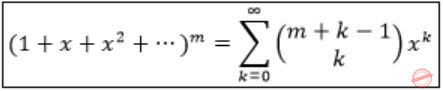Here, m=3, k=3, the coefficient= 5C3 = 5!/2!3! = 10
 Question 47

Consider the recurrence relation a1 = 8, an = 6n2 + 2n + an-1. Let a99 = K × 104. The value of K is ___________.

 A 198 B 199 C 200 D 201
Engineering-Mathematics       Combinatorics       GATE 2016 [Set-1]       Video-Explanation
Question 47 Explanation:
an = 6n2 + 2n + a(n-1)
Replace a(n-1)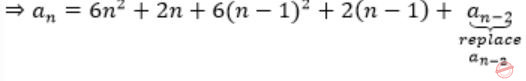⇒ an = 6n2 + 2n + 6(n-1)2 + 2(n-1) + 6(n-2)2 + 2(n-2) + ⋯ a1
Given that a1 = 8, replace it
⇒ an = 6n2 + 2n + 6(n-1)2 + 2(n-1) + 6(n-2)2 + 2(n-2) + ⋯8
= 6n2 + 2n + 6(n-1)2 + 2(n-1) + 6(n-2)2 + 2(n-2) + ⋯ + 6(1)2 + 2(1)= 6(n2 + (n-1)2 + (n-2)2 + ⋯ + 22 + 12) + 2(n + (n-1) + ⋯1)
Sum of n2 = (n(n+1)(2n+1))/6
Sum of n = (n(n+1))/2
= 6 × (n(n+1)(2n+1))/6 + 2×(n(n+1))/2
= n(n+1)[1+2n+1]
= n(n+1)[2n+2]
= 2n(n+1)2
Given a99 = k×104
a99 = 2(99)(100)2 = 198 × 104
∴k = 198
 Question 48
A function f:N+ → N+, deﬁned on the set of positive integers N+, satisﬁes the following properties:
f(n) = f(n/2)           if n is even
f(n) = f(n+5)           if n is odd
Let R = {i|∃j: f(j)=i} be the set of distinct values that f takes. The maximum possible size of R is __________.
 A 2 B 3 C 4 D 5
Engineering-Mathematics       Set-Theory       GATE 2016 [Set-1]       Video-Explanation
Question 48 Explanation:
f(n)= f(n⁄2)          if n is even
f(n)= f(n+5)        if n is oddWe can observe thatand f(5) = f(10) = f(15) = f(20)
Observe that f(11) = f(8)
f(12) = f(6) = f(3)
f(13) = f(9) = f(14) = f(7) = f(12) = f(6) = f(3)
f(14) = f(9) = f(12) = f(6) = f(3)
f(16) = f(8) = f(4) = f(2) = f(1) [repeating]
So, we can conclude that
‘R’ can have size only ‘two’ [one: multiple of 5’s, other: other than 5 multiples]
 Question 49
Consider the following experiment.
Step1. Flip a fair coin twice.
Step2. If the outcomes are (TAILS, HEADS) then output Y and stop.
Step4. If the outcomes are (TAILS, TAILS), then go to Step 1.
The probability that the output of the experiment is Y is (up to two decimal places) ________.
 A 0.33 B 0.34 C 0.35 D 0.36
Engineering-Mathematics       Probability       GATE 2016 [Set-1]       Video-Explanation
Question 49 Explanation:
If a coin is flipped twice, the possible outcomes {HH, HT, TH, TT}
Stop conditions:
If outcome = TH then Stop [output 4] ————— (1)
else
outcome = HH/ HT then Stop [output N] ————– (2)
We get ‘y’ when we have (1) i.e., ‘TH’ is output.
(1) can be preceded by ‘TT’ also, as ‘TT’ will reset (1) again
Probability of getting y = TH + (TT)(TH) + (TT)(TT)(TH) + …
= 1/2 × 1/2 + 1/2 × 1/2 × 1/2 × 1/2 + …
= (1/4)/(1-1/4)
= 1/3
= 0.33
 Question 50
Consider the following expressions:
(i) false
(ii) Q
(iii) true
(iv) P ∨ Q
(v) ¬Q ∨ P
The number of expressions given above that are logically implied by P ∧ (P ⇒ Q) is _________.
 A 4 B 5 C 6 D 7
Engineering-Mathematics       Propositional-Logic       GATE 2016 [Set-2]       Video-Explanation
Question 50 Explanation:
The expression is logically implied by P ∧ (P → Q) means
(P ∧ (P → Q))→ expression is a tautology. So we have to find
How many tautological formulas are there for the given inputs.
(P ∧ (P → Q)) → True is always tautology
(P ∧ (P → Q)) → False is not a tautology
(P ∧ (P → Q)) → Q is a tautology
(P ∧ (P → Q)) → ¬Q ∨ P is a tautology
(P ∧ (P → Q)) → P ∨ Q is a tautology
So there are 4 expressions logically implied by (P ∧ (P → Q))
There are 50 questions to complete.

Register Now